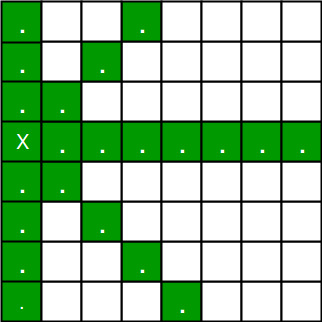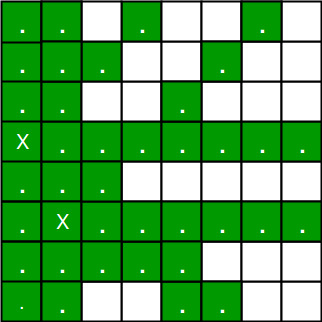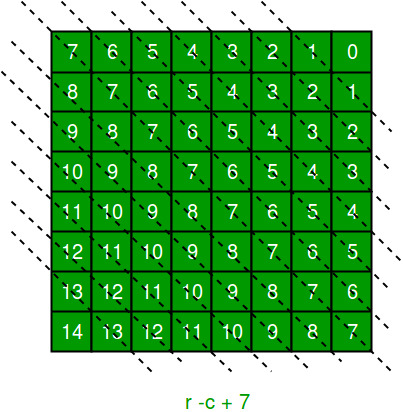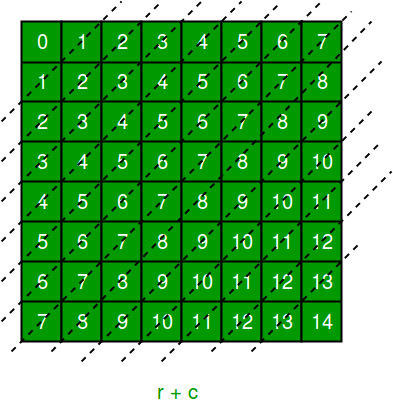# N Queen Problem using Branch And Bound

The N queens puzzle is the problem of placing N chess queens on an N×N chessboard so that no two queens threaten each other. Thus, a solution requires that no two queens share the same row, column, or diagonal.

Backtracking Algorithm for N-Queen is already discussed here. In backtracking solution we backtrack when we hit a dead end. In Branch and Bound solution, after building a partial solution, we figure out that there is no point going any deeper as we are going to hit a dead end.

Let’s begin by describing backtracking solution. “The idea is to place queens one by one in different columns, starting from the leftmost column. When we place a queen in a column, we check for clashes with already placed queens. In the current column, if we find a row for which there is no clash, we mark this row and column as part of the solution. If we do not find such a row due to clashes, then we backtrack and return false.”1. For the 1st Queen, there are total 8 possibilities as we can place 1st Queen in any row of first column. Let’s place Queen 1 on row 3.
2. After placing 1st Queen, there are 7 possibilities left for the 2nd Queen. But wait, we don’t really have 7 possibilities. We cannot place Queen 2 on rows 2, 3 or 4 as those cells are under attack from Queen 1. So, Queen 2 has only 8 – 3 = 5 valid positions left.
3. After picking a position for Queen 2, Queen 3 has even fewer options as most of the cells in its column are under attack from the first 2 Queens.

We need to figure out an efficient way of keeping track of which cells are under attack. In previous solution we kept an 8­-by­-8 Boolean matrix and update it each time we placed a queen, but that required linear time to update as we need to check for safe cells.

Basically, we have to ensure 4 things:
1. No two queens share a column.
2. No two queens share a row.
3. No two queens share a top-right to left-bottom diagonal.
4. No two queens share a top-left to bottom-right diagonal.

Number 1 is automatic because of the way we store the solution. For number 2, 3 and 4, we can perform updates in O(1) time. The idea is to keep three Boolean arrays that tell us which rows and which diagonals are occupied.

Lets do some pre-processing first. Let’s create two N x N matrix one for / diagonal and other one for \ diagonal. Let’s call them slashCode and backslashCode respectively. The trick is to fill them in such a way that two queens sharing a same /­diagonal will have the same value in matrix slashCode, and if they share same \­diagonal, they will have the same value in backslashCode matrix.

For an N x N matrix, fill slashCode and backslashCode matrix using below formula –

slashCode[row][col] = row + col
backslashCode[row][col] = row – col + (N-1)

Using above formula will result in below matricesThe ‘N – 1’ in the backslash code is there to ensure that the codes are never negative because we will be using the codes as indices in an array.

Now before we place queen i on row j, we first check whether row j is used (use an array to store row info). Then we check whether slash code ( j + i ) or backslash code ( j – i + 7 ) are used (keep two arrays that will tell us which diagonals are occupied). If yes, then we have to try a different location for queen i. If not, then we mark the row and the two diagonals as used and recurse on queen i + 1. After the recursive call returns and before we try another position for queen i, we need to reset the row, slash code and backslash code as unused again, like in the code from the previous notes.

Below is the implementation of above idea –

## C++

 `/* C++ program to solve N Queen Problem using Branch ` `   ``and Bound */` `#include ` `#include ` `#define N 8 ` ` `  `/* A utility function to print solution */` `void` `printSolution(``int` `board[N][N]) ` `{ ` `    ``for` `(``int` `i = 0; i < N; i++) ` `    ``{ ` `        ``for` `(``int` `j = 0; j < N; j++) ` `            ``printf``(``"%2d "``, board[i][j]); ` `        ``printf``(``"\n"``); ` `    ``} ` `} ` ` `  `/* A Optimized function to check if a queen can ` `be placed on board[row][col] */` `bool` `isSafe(``int` `row, ``int` `col, ``int` `slashCode[N][N], ` `            ``int` `backslashCode[N][N], ``bool` `rowLookup[], ` `            ``bool` `slashCodeLookup[], ``bool` `backslashCodeLookup[] ) ` `{ ` `    ``if` `(slashCodeLookup[slashCode[row][col]] || ` `        ``backslashCodeLookup[backslashCode[row][col]] || ` `        ``rowLookup[row]) ` `    ``return` `false``; ` ` `  `    ``return` `true``; ` `} ` ` `  `/* A recursive utility function to solve N Queen problem */` `bool` `solveNQueensUtil(``int` `board[N][N], ``int` `col, ` `    ``int` `slashCode[N][N], ``int` `backslashCode[N][N], ``bool` `rowLookup[N], ` `    ``bool` `slashCodeLookup[], ``bool` `backslashCodeLookup[] ) ` `{ ` `    ``/* base case: If all queens are placed ` `    ``then return true */` `    ``if` `(col >= N) ` `        ``return` `true``; ` ` `  `    ``/* Consider this column and try placing ` `       ``this queen in all rows one by one */` `    ``for` `(``int` `i = 0; i < N; i++) ` `    ``{ ` `        ``/* Check if queen can be placed on ` `           ``board[i][col] */` `        ``if` `( isSafe(i, col, slashCode, backslashCode, rowLookup, ` `                    ``slashCodeLookup, backslashCodeLookup) ) ` `        ``{ ` `            ``/* Place this queen in board[i][col] */` `            ``board[i][col] = 1; ` `            ``rowLookup[i] = ``true``; ` `            ``slashCodeLookup[slashCode[i][col]] = ``true``; ` `            ``backslashCodeLookup[backslashCode[i][col]] = ``true``; ` ` `  `            ``/* recur to place rest of the queens */` `            ``if` `( solveNQueensUtil(board, col + 1, slashCode, backslashCode, ` `                    ``rowLookup, slashCodeLookup, backslashCodeLookup) ) ` `                ``return` `true``; ` ` `  `            ``/* If placing queen in board[i][col] ` `            ``doesn't lead to a solution, then backtrack */` ` `  `            ``/* Remove queen from board[i][col] */` `            ``board[i][col] = 0; ` `            ``rowLookup[i] = ``false``; ` `            ``slashCodeLookup[slashCode[i][col]] = ``false``; ` `            ``backslashCodeLookup[backslashCode[i][col]] = ``false``; ` `        ``} ` `    ``} ` ` `  `    ``/* If queen can not be place in any row in ` `        ``this colum col then return false */` `    ``return` `false``; ` `} ` ` `  `/* This function solves the N Queen problem using ` `Branch and Bound. It mainly uses solveNQueensUtil() to ` `solve the problem. It returns false if queens ` `cannot be placed, otherwise return true and ` `prints placement of queens in the form of 1s. ` `Please note that there may be more than one ` `solutions, this function prints one of the ` `feasible solutions.*/` `bool` `solveNQueens() ` `{ ` `    ``int` `board[N][N]; ` `    ``memset``(board, 0, ``sizeof` `board); ` ` `  `    ``// helper matrices ` `    ``int` `slashCode[N][N]; ` `    ``int` `backslashCode[N][N]; ` ` `  `    ``// arrays to tell us which rows are occupied ` `    ``bool` `rowLookup[N] = {``false``}; ` ` `  `    ``//keep two arrays to tell us which diagonals are occupied ` `    ``bool` `slashCodeLookup[2*N - 1] = {``false``}; ` `    ``bool` `backslashCodeLookup[2*N - 1] = {``false``}; ` ` `  `    ``// initialize helper matrices ` `    ``for` `(``int` `r = 0; r < N; r++) ` `        ``for` `(``int` `c = 0; c < N; c++) ` `            ``slashCode[r] = r + c, ` `            ``backslashCode[r] = r - c + 7; ` ` `  `    ``if` `(solveNQueensUtil(board, 0, slashCode, backslashCode, ` `      ``rowLookup, slashCodeLookup, backslashCodeLookup) == ``false` `) ` `    ``{ ` `        ``printf``(``"Solution does not exist"``); ` `        ``return` `false``; ` `    ``} ` ` `  `    ``// solution found ` `    ``printSolution(board); ` `    ``return` `true``; ` `} ` ` `  `// driver program to test above function ` `int` `main() ` `{ ` `    ``solveNQueens(); ` ` `  `    ``return` `0; ` `} `

## Python3

 `""" Python3 program to solve N Queen Problem  ` `using Branch or Bound """` ` `  `N ``=` `8` ` `  `""" A utility function to prsolution """` `def` `printSolution(board): ` `    ``for` `i ``in` `range``(N): ` `        ``for` `j ``in` `range``(N): ` `            ``print``(board[i][j], end ``=` `" "``) ` `        ``print``() ` ` `  `""" A Optimized function to check if  ` `a queen can be placed on board[row][col] """` `def` `isSafe(row, col, slashCode, backslashCode,  ` `           ``rowLookup, slashCodeLookup, backslashCodeLookup): ` `    ``if` `(slashCodeLookup[slashCode[row][col]] ``or` `        ``backslashCodeLookup[backslashCode[row][col]] ``or` `        ``rowLookup[row]): ` `        ``return` `False` `    ``return` `True` ` `  `""" A recursive utility function to solve N Queen problem """` `def` `solveNQueensUtil(board, col, slashCode, backslashCode,  ` `                     ``rowLookup, slashCodeLookup, ` `                     ``backslashCodeLookup): ` `                         `  `    ``""" base case: If all queens are placed then return True """` `    ``if``(col >``=` `N): ` `        ``return` `True` `    ``for` `i ``in` `range``(N): ` `        ``if``(isSafe(i, col, slashCode, backslashCode,  ` `                  ``rowLookup, slashCodeLookup, ` `                  ``backslashCodeLookup)): ` `                     `  `            ``""" Place this queen in board[i][col] """` `            ``board[i][col] ``=` `1` `            ``rowLookup[i] ``=` `True` `            ``slashCodeLookup[slashCode[i][col]] ``=` `True` `            ``backslashCodeLookup[backslashCode[i][col]] ``=` `True` `             `  `            ``""" recur to place rest of the queens """` `            ``if``(solveNQueensUtil(board, col ``+` `1``, slashCode, backslashCode,  ` `                                ``rowLookup, slashCodeLookup,  ` `                                ``backslashCodeLookup)): ` `                ``return` `True` `             `  `            ``""" If placing queen in board[i][col]  ` `            ``doesn't lead to a solution,then backtrack """` `             `  `            ``""" Remove queen from board[i][col] """` `            ``board[i][col] ``=` `0` `            ``rowLookup[i] ``=` `False` `            ``slashCodeLookup[slashCode[i][col]] ``=` `False` `            ``backslashCodeLookup[backslashCode[i][col]] ``=` `False` `             `  `    ``""" If queen can not be place in any row in  ` `    ``this colum col then return False """` `    ``return` `False` ` `  `""" This function solves the N Queen problem using  ` `Branch or Bound. It mainly uses solveNQueensUtil()to  ` `solve the problem. It returns False if queens  ` `cannot be placed,otherwise return True or  ` `prints placement of queens in the form of 1s.  ` `Please note that there may be more than one  ` `solutions,this function prints one of the  ` `feasible solutions."""` `def` `solveNQueens(): ` `    ``board ``=` `[[``0` `for` `i ``in` `range``(N)]  ` `                ``for` `j ``in` `range``(N)] ` `     `  `    ``# helper matrices  ` `    ``slashCode ``=` `[[``0` `for` `i ``in` `range``(N)]  ` `                    ``for` `j ``in` `range``(N)] ` `    ``backslashCode ``=` `[[``0` `for` `i ``in` `range``(N)]  ` `                        ``for` `j ``in` `range``(N)] ` `     `  `    ``# arrays to tell us which rows are occupied  ` `    ``rowLookup ``=` `[``False``] ``*` `N ` `     `  `    ``# keep two arrays to tell us  ` `    ``# which diagonals are occupied  ` `    ``x ``=` `2` `*` `N ``-` `1` `    ``slashCodeLookup ``=` `[``False``] ``*` `x ` `    ``backslashCodeLookup ``=` `[``False``] ``*` `x ` `     `  `    ``# initialize helper matrices  ` `    ``for` `rr ``in` `range``(N): ` `        ``for` `cc ``in` `range``(N): ` `            ``slashCode[rr][cc] ``=` `rr ``+` `cc ` `            ``backslashCode[rr][cc] ``=` `rr ``-` `cc ``+` `7` `     `  `    ``if``(solveNQueensUtil(board, ``0``, slashCode, backslashCode,  ` `                        ``rowLookup, slashCodeLookup,  ` `                        ``backslashCodeLookup) ``=``=` `False``): ` `        ``print``(``"Solution does not exist"``) ` `        ``return` `False` `         `  `    ``# solution found  ` `    ``printSolution(board) ` `    ``return` `True` ` `  `# Driver Cde ` `solveNQueens() ` ` `  `# This code is contributed by SHUBHAMSINGH10 `

Output :

``` 1  0  0  0  0  0  0  0
0  0  0  0  0  0  1  0
0  0  0  0  1  0  0  0
0  0  0  0  0  0  0  1
0  1  0  0  0  0  0  0
0  0  0  1  0  0  0  0
0  0  0  0  0  1  0  0
0  0  1  0  0  0  0  0 ```

Performance:
When run on local machines for N = 32, the backtracking solution took 659.68 seconds while above branch and bound solution took only 119.63 seconds. The difference will be even huge for larger values of N.Attention reader! Don’t stop learning now. Get hold of all the important DSA concepts with the DSA Self Paced Course at a student-friendly price and become industry ready.

My Personal Notes arrow_drop_up

Article Tags :

2

Please write to us at contribute@geeksforgeeks.org to report any issue with the above content.